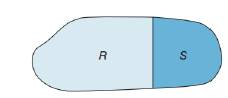Chapter 8.1, Problem 43EElementary Geometry For College St...

7th Edition
Alexander + 2 others
ISBN: 9781337614085

Solutions

Chapter
SectionElementary Geometry For College St...

7th Edition
Alexander + 2 others
ISBN: 9781337614085
Textbook Problem

Given region R ∪ S , explain why A R ∪ S > A R .To determine

To explain:

The region ARS>AR if the region RS.

Explanation

Given,

From the figure, region R and S are the mutually exclusive and exhaustive.

(i.e.) RS

Where RS=R+S

Area of the region R and S is AR and AS.

Thus, the area of the figure is ARS

(i.e.) ARS=AR+S

Still sussing out bartleby?

Check out a sample textbook solution.

See a sample solution

The Solution to Your Study Problems

Bartleby provides explanations to thousands of textbook problems written by our experts, many with advanced degrees!

Get Started

Given Q=CV,C=12, and V=2500, find Q.

Elementary Technical Mathematics

Graphing Inequalities Graph the inequality. 98. y x2 3

Precalculus: Mathematics for Calculus (Standalone Book)

Evaluate the limit, if it exists. limx164x16xx2

Single Variable Calculus: Early Transcendentals

In Exercises 1-22, evaluate the given expression. P(5,3)

Finite Mathematics for the Managerial, Life, and Social Sciences

Write in cylindrical coordinates, where E is the solid at the right.

Study Guide for Stewart's Multivariable Calculus, 8th